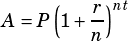For #23 on Test 8, I understand the “q/4” part on the exponent but why does the rate stay the same? Wouldn’t you divide 1.02 by 4?

First, here’s my general solution for this one (the link in the test explations page wasn’t working before for some reason).

If I’m understanding your question right, you’re thinking about the standard compound interest formula,where r is the interest rate, n is the number of times interest is applied per period, and t is the number of periods.

The difference here is that we’re not actually changing the frequency of compounding in the model. All we’re doing is converting years, t, to quarters. In other words, the number of times the growth compounds over a year doesn’t change, we’re just thinking about years in smaller chunks.

Does that help?